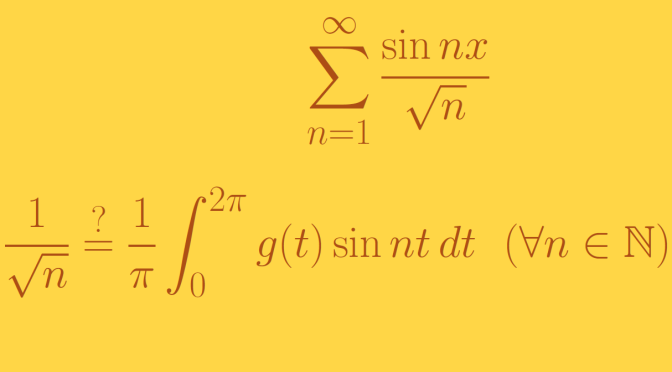A trigonometric series that is not a Fourier series (Riemann-integration)

We’re looking here at convergent trigonometric series like $f(x) = a_0 + \sum_{k=1}^\infty (a_n \cos nx + b_n \sin nx)$ which are convergent but are not Fourier series. Which means that the terms $$a_n$$ and $$b_n$$ cannot be written$\begin{array}{ll} a_n = \frac{1}{\pi} \int_0^{2 \pi} g(t) \cos nt \, dt & (n= 0, 1, \dots) \\ b_n = \frac{1}{\pi} \int_0^{2 \pi} g(t) \sin nt \, dt & (n= 1, 2, \dots) \end{array}$ where $$g$$ is any integrable function.

This raises the question of the type of integral used. We cover here an example based on Riemann integral. I’ll cover a Lebesgue integral example later on.

We prove here that the function $f(x)= \sum_{n=1}^\infty \frac{\sin nx}{\sqrt{n}}$ is a convergent trigonometric series but is not a Fourier series.

$$f$$ is a convergent trigonometric series on $$[0,2 \pi]$$

$$f$$ is periodic with periodicity $$2 \pi$$. $$f$$ is also odd as the sine function is. Hence we can study the function $$f$$ on $$[0, \pi]$$ only. The series is convergent at $$0$$ as all the terms of the series defining $$f$$ are vanishing at $$0$$.

The sum $\widetilde{D}_n(x) = \sum_{k=1}^n \sin kx$ is the imaginary part of $1+z+ z^2+ \dots +z^n=\frac{1-z^{n+1}}{1-z}$ where $$z=e^{ix}$$. Hence for $$0< \epsilon <\pi$$ we have $\widetilde{D}_n(x) = \sum_{k=1}^n \sin kx = \frac{\cos \frac{x}{2} - \cos (n+\frac{1}{2})x}{2 \sin \frac{x}{2}}$ for all $$x \in [\epsilon,\pi]$$. Therefore $$\widetilde{D}_n(x)$$ is uniformly bounded by $$\frac{1}{\sin \frac{\epsilon}{2}}$$ on the interval $$[\epsilon,\pi]$$. Applying an Abel transformation we get $\left\vert \sum_{k=n}^\infty \frac{\sin kx}{\sqrt{n}} \right\vert \le \frac{2}{\sqrt{n} \sin \frac{\epsilon}{2}}$ for all $$x \in [\epsilon,\pi]$$. This implies that the trigonometric series $$\sum_{n=1}^\infty \frac{\sin nx}{\sqrt{n}}$$ is uniformly convergent on all closed intervals $$[\epsilon,\pi]$$ where $$0< \epsilon <\pi$$. In particular, $$f$$ is defined everywhere and continuous on $$(0,\pi]$$.

$$f$$ is not a Fourier series

If the function $$f$$ was the Fourier series of any Riemann-integrable function $$g$$, $$g^2$$ would also be Riemann-integrable. By Bessel’s inequality, we would have $\sum_{n=1}^\infty \left(\frac{1}{\sqrt{n}} \right)^2 = \sum_{n=1}^\infty \frac{1}{n} \le \frac{1}{\pi} \int_0^{2 \pi} g^2(t) \, dt$ Which cannot be as the harmonic series $$\sum \frac{1}{n}$$ diverges.